Study of mathematics online.
Study math with us and make sure that "Mathematics is easy!"

# Volume Formulas for Geometric Shapes

Volume
is the amount of space that an object occupies; measured in units cubed.

## Volume of a Cube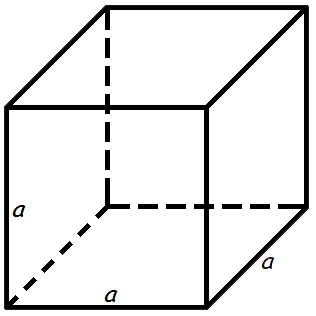The volume of a Cube is equal to the cube of the length of its edge.

Volume formula of a Cube:

V = a3
where V - a cube volume,
a - the length of an edge.

## Volume of a Prism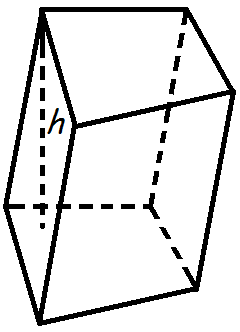The volume of a prism is equal to the product of the base area to a height of a prism.

Volume formula of a prism:

V = Ab h
where V - volume of the prism,
Ab - the area of the base of the prism,
h - the height of the prism.

## Volume of a parallelepiped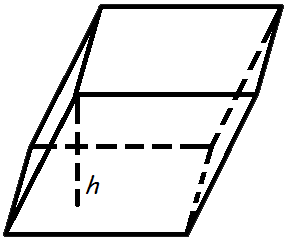The volume of a parallelepiped is equal to the product of the base area to a height of a parallelepiped.

Volume formula of a parallelepiped:

V = Ab · h
where V - volume of the parallelepiped,
Ab - the area of the base of the parallelepiped,
h - the height of the parallelepiped.

## Volume of a Rectangular Prism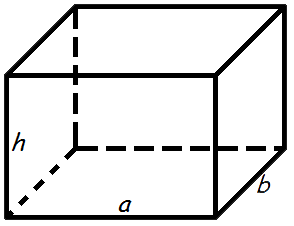The volume of a rectangular prism is equal to the product of its length, width and height.

Volume formula of a rectangular prism:

V = a · b · h
where V - volume of a rectangular prism,
a - length,
b - width,
h - height.

## Volume of a Pyramid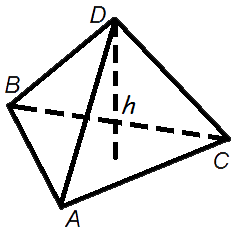The volume of a pyramid is equal to one third of the product of the area of its base times the height.

The volume formula of a pyramid:

 V = 1 Ab · h 3
where V - the volume of a pyramid,
Ab - the area of the pyramid base,
h - the height of the pyramid.

## Volume of a Tetrahedron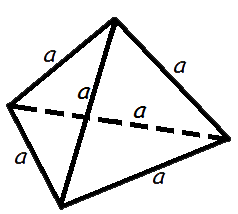Volume formulas of a tetrahedron:

 V = a3√2 12
where V - volume of a tetrahedron,
a - edge length.

## Volume of a Right Circular Cylinder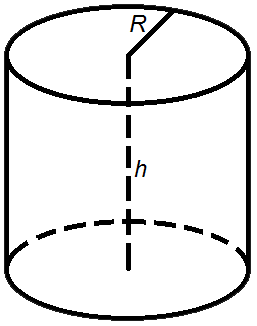Volume of a right circular cylinder is equal to the product of the area of its base times the height.

Volume formula of a right circular cylinder:
V = π R2 h
V = Ab h
where V - volume of a cylinder,
Ab - area of the base,
R - radius of the base,
h - height,
π = 3.141592.

## Volume of a Right Circular Cone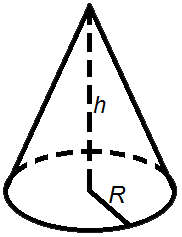Volume of a right circular cone is equal to one third of the product of the area of its base times the height.

Volume formula of a right circular cone:

 V = 1 π R2 h 3
 V = 1 Ab h 3
where V - volume of a cone,
Ab - area of the base,
R - radius of the base,
h - height,
π = 3.141592.

## Volume of a Sphere (Solid)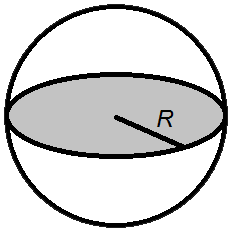Volume of a sphere is equal to four-thirds of its radius cubed multiplied by the number pi.

Volume formula of a sphere (solid):

 V = 4 π R3 3
where V - volume of a sphere,
R - radius of a sphere,
π = 3.141592.